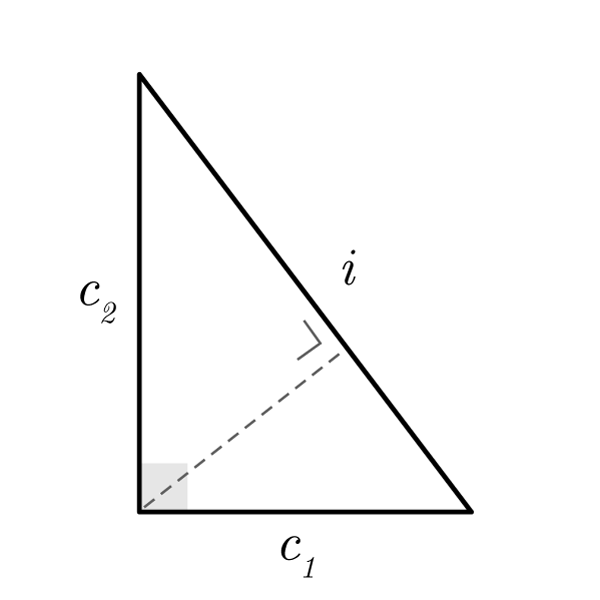# Right Triangle

 Minimum Cathetus $$c_{1}$$ Maximum Cathetus $$c_{2}$$ Hypotenuse $$i$$ Height $$h$$ Min Projection $$p_{1}$$ Max Projection $$p_{2}$$
$$2p = i + c_{1} + c_{2}$$
Perimeter
$$A = \frac{c_{1} \times c_{2}}{2}$$
Area
$$c_{1} = \frac{A \times 2}{c_{2}}$$
$$c_{2} = \frac{A \times 2}{c_{1}}$$
$$A = \frac{i \times h}{2}$$
Area
$$i = \frac{A \times 2}{h}$$
$$h = \frac{A \times 2}{i}$$
Pythagoras' theorem
$$i = \sqrt{ {c_{1}}^2 + {c_{2}}^2 }$$
Pythagoras' theorem
$$c_{1} = \sqrt{ {i}^2 - {c_{2}}^2 }$$
$$c_{2} = \sqrt{ {i}^2 - {c_{1}}^2 }$$
$$i : c_{1} = c_{1} : p_{1}$$
$$i : c_{2} = c_{2} : p_{2}$$
$$p_{1} : h = h : p_{2}$$
Isosceles Right Triangle
$$i = c \sqrt{2}$$
$$c = \frac{i}{\sqrt{2}}$$
$$A = \frac{{c}^2}{2}$$
$$A = \frac{{i}^2}{4}$$
$$c = \sqrt{2A}$$
Right Triangle 30, 60, 90°
$$i = c_{1} \times 2$$
Hypotenuse
$$c_{1} = \frac{i}{2}$$
Minimum Cathetus
$$c_{2} = c_{1} \sqrt{3}$$
Maximum Cathetus
$$c_{1} = \frac{c_{2}}{\sqrt{3}}$$
Minimum Cathetus
$$c_{2} = i \frac{\sqrt{3}}{2}$$
Maximum Cathetus
$$i = c_{2} \frac{2}{\sqrt{3}}$$
Hypotenuse

## Definition

The right triangle is a triangle with a right angle (90 degree).

## Properties

1. It has a right angle (90 degree)
2. The Pythagoras' theorem is valid
3. All the  Generic Triangle formulas are valid
4. It can be inscribed inside a semicircle where the radius is half the hypotenuse## Right Triangle Formulas

Data Formula
Perimeter 2p = i + c1 + c2
Area A = (c1 × c2) / 2
Area A = (i × h) / 2
Hypotenuse i = √( c12 + c22 )
Minimum Cathetus c1 = √( i2 - c22 )
Maximum Cathetus c2 = √( i2 - c12 )
Minimum Cathetus c1 = (A × 2) / c2
Maximum Cathetus c2 = (A × 2) / c1
Hypotenuse i = (A × 2) / h
Height h = (A × 2) / i

## Isosceles Right Triangle Formulas

Data Formula
Hypotenuse i = c √2
Cathetus c = i / (√2)
Area A = c2 / 2
Area A = i2 / 4
Cathetus c = √(2A)

## Right Triangle 30, 60, 90° Formulas

Data Formula
Hypotenuse i = c1 × 2
Minimum Cathetus c1 = i / 2
Maximum Cathetus c2 = c1 √3
Minimum Cathetus c1 = c2 / (√3)
Maximum Cathetus c2 = i (√3 / 2)
Hypotenuse i = c2 (2 / √3)